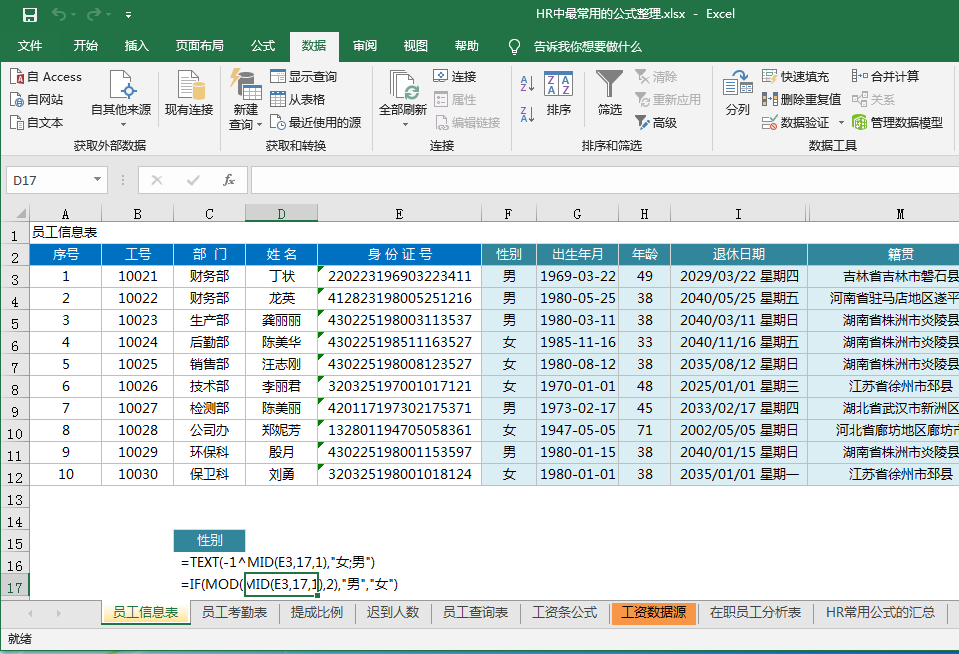# 适合的Excel公式大全1、计算性别(F列)

=IF(MOD(MID(E3,17,1),2),"男","女")

2、出生年月(G列)

=TEXT(MID(E3,7,8),"0-00-00")

3、年龄公式(H列)

=datedif(G3,TODAY(),"y")

4、退休日期 (I列)

=TEXT(EDATE(G3,12*(5*(F3="男")+55)),"yyyy/mm/dd aaaa")

5、籍贯(M列)

=VLOOKUP(LEFT(E3,6)*1,地址库!E:F,2,)

6、社会工龄(T列)

=DATEDIF(S3,NOW(),"y")

7、公司工龄(W列)

=DATEDIF(V3,NOW(),"y")&"年"&DATEDIF(V3,NOW(),"ym")&"月"&DATEDIF(V3,NOW(),"md")&"天"

8、合同续签日期(Y列)

=DATE(YEAR(V3)+LEFTB(X3,2),MONTH(V3),DAY(V3))-1

9、合同到期日期(Z列)

=TEXT(EDATE(V3,LEFTB(X3,2)*12)-TODAY(),"[<0]过期0天;[<30]即将到期0天;还早")

10、工龄工资(AA列)

=MIN(700,DATEDIF(\$V3,NOW(),"y")*50)

11、生肖(AB列)

=MID("猴鸡狗猪鼠牛虎兔龙蛇马羊",MOD(MID(E3,7,4),12)+1,1)

1、本月工作日天数(AG列)

=NETWORKDAYS(B\$5,DATE(YEAR(N\$4),MONTH(N\$4)+1,),)

2、调休天数公式(AI列)

=countif(B9:AE9,"调")

3、扣钱公式(AO列)

=SUM((B9:AE9={"事";"旷";"病";"丧";"婚"})*{30;50;20;10;10})

1、本科学历人数

=COUNTIF(D:D,"本科")

2、办公室本科学历人数

=COUNTIFS(A:A,"办公室",D:D,"本科")

3、30~40岁总人数

=COUNTIFS(F:F,">=30",F:F,"<40")

1、提成比率计算

=VLOOKUP(B3,\$C\$12:\$E\$21,3)

2、个人所得税计算

=5*MAX(A2*{0.6,2,4,5,6,7,9}%-{21,91,251,376,761,1346,3016},)

3、工资条公式

=CHOOSE(MOD(ROW(A3),3)+1,工资数据源!A\$1,OFFSET(工资数据源!A\$1,INT(ROW(A3)/3),,),"")

· A3:标题行的行数+2,如果标题行在第3行，则A3改为A5

· 工资数据源!A\$1:工资表的标题行的第一列位置

4、CountIF函数统计身份证号码出错的解决方法

=Countif(A:A,A2&"*")

1、各部门人数占比

2、各个年龄段人数和占比

3、各个部门各年龄段占比

4、各部门学历统计

5、按年份统计各部门入职人数

# 附：HR工作中常用分析公式

1.【新进员工比率】＝已转正员工数/在职总人数

2.【补充员工比率】＝为离职缺口补充的人数/在职总人数

3.【离职率】（主动离职率/淘汰率＝离职人数/在职总人数=离职人数/(期初人数+录用人数)×100％

4.【异动率】＝异动人数/在职总人数

5.【人事费用率】＝（人均人工成本*总人数）/同期销售收入总数

6.【招聘达成率】=（报到人数+待报到人数）/（计划增补人数+临时增补人数）